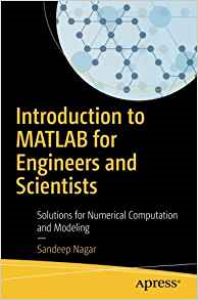# Introduction to MATLAB for Engineers and Scientists: Solutions for Numerical Computation and Modeling (English Edition) Format Kindle

PLDZ-17 In Stock
\$ 5.00 USD
Description
Familiarize yourself with MATLAB using this concise, practical tutorial that is focused on writing code to learn concepts. Starting from the basics, this book covers array-based computing, plotting and working with files, numerical computation formalism, and the primary concepts of approximations. Introduction to MATLAB is useful for industry engineers, researchers, and students who are looking for open-source solutions for numerical computation.

In this book you will learn by doing, avoiding technical jargon, which makes the concepts easy to learn. First you’ll see how to run basic calculations, absorbing technical complexities incrementally as you progress toward advanced topics. Throughout, the language is kept simple to ensure that readers at all levels can grasp the concepts.

What You'll Learn
• Apply sample code to your engineering or science problems
• Work with MATLAB arrays, functions, and loops
• Use MATLAB’s plotting functions for data visualization
• Solve numerical computing and computational engineering problems with a MATLAB case study

Who This Book Is For

Engineers, scientists, researchers, and students who are new to MATLAB. Some prior programming experience would be helpful but not required.

Recent Reviews Write a Review
0 0 0 0 reviews